# Quality criteria

## 1. Winding factors

For additional information, refer to section dedicated to the winding factor since it’s the same as Classical winding topology.

For unbalanced hairpin configurations, as the results are not relevant, therefore, they are not computed and displayed.

Note: The unbalanced hairpin configurations are characterized by at least one parallel path which is different in term of voltage and impedance from the other parallel paths.

## 2. Slot star

The Slot star represents the total vectorial sum of voltages, at the ends of each coil, for each parallel path.

A slot star is computed and displayed for each parallel path.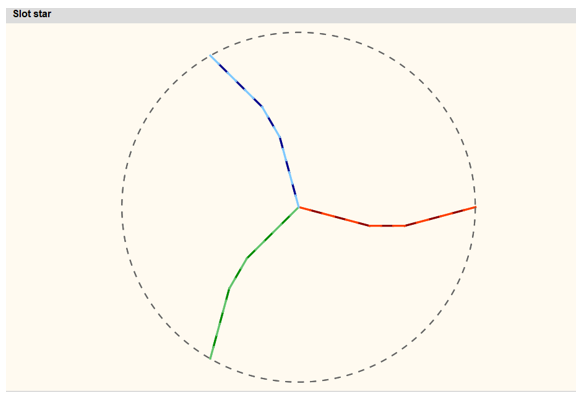Slot star - Strong or weak balance case example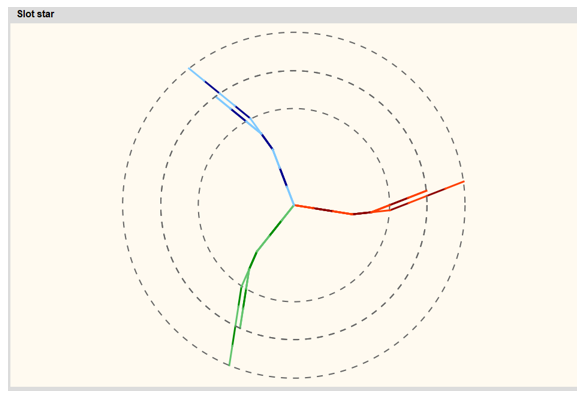Slot star - Unbalance case example 1 Balance analysis classification: - When the hairpin configuration is balanced (strong and weak balance), all the slot stars are superimposed (to differentiate strong and weak balance case referred to the table Parallel paths) When the hairpin configuration is unbalanced, there are as many different slot stars (circles) as there are different unbalanced parallel paths
Note: Definition of Strong and weak balance are done into the section

## 3. Parallel paths

For each slot per pole and per phase of each parallel path, the number of conductors in each conductor layer is computed and displayed in a table

The three kinds of possible configurations in term of electrical current in parallel paths are illustrated below: Strong balance, weak balance and unbalance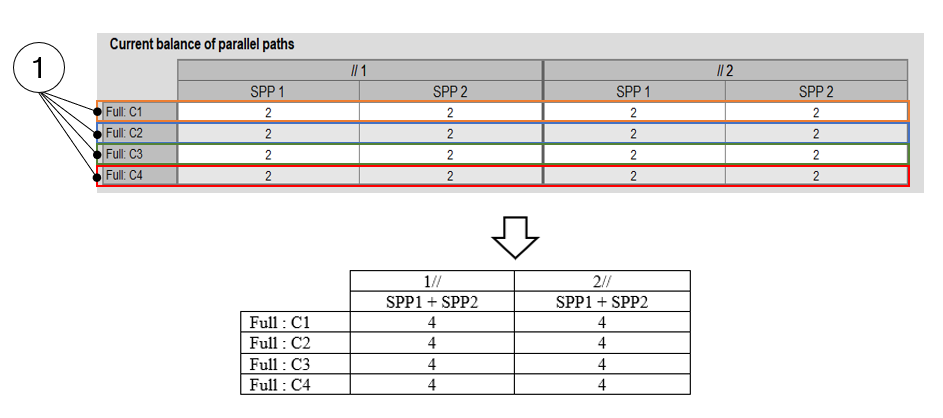Current balance of parallel paths – Strong balance case example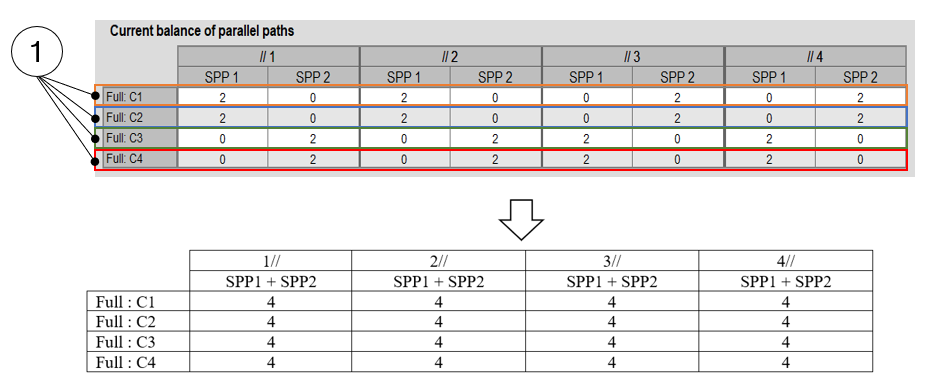Current balance of parallel paths – Weak balance case example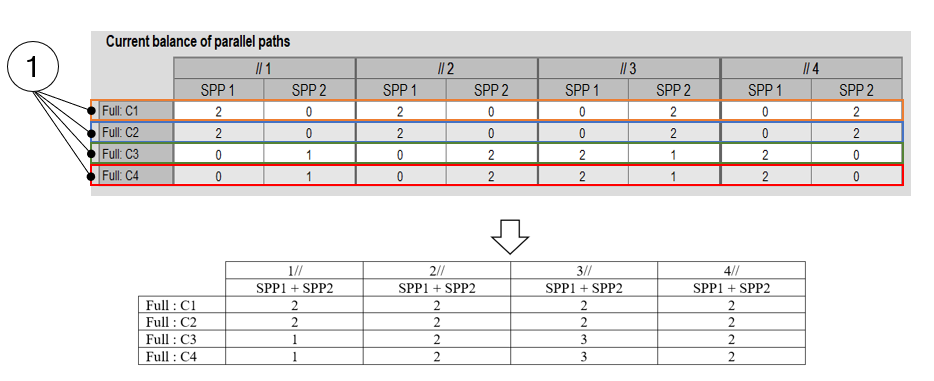Current balance of parallel paths – Unbalance case example 1 Layer of conductors 2 Balance analysis classification: - When the same number of conductors are displayed in all the cells, a “strong balance hairpin configuration” is obtained. This is the best winding design configuration. If for each parallel path and all layers of conductors the sums of conductors are the same, a “weak balance hairpin configuration” is probably obtained (to be confirmed with the slot star if all the circles are well superimposed). If for each parallel path and all layers of conductors the sums of conductors are different, an “unbalance hairpin configuration” is obtained.

## 4. Voltage drop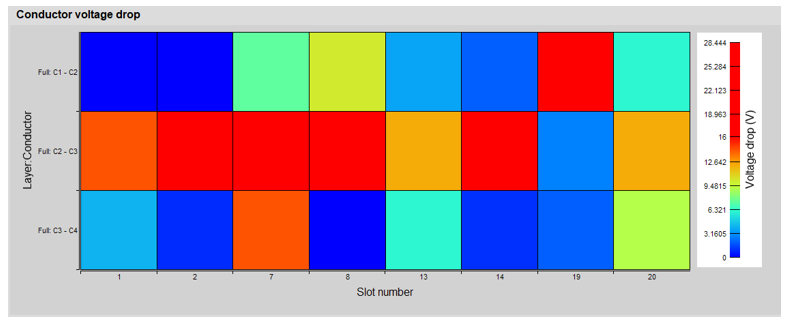Conductor voltage drop 1 Inside each slot, the voltage drop between the superimposed conductors for calculating the maximum Line-Line voltage value and the voltage drop limit set by the user (X-factor: model evaluation table). This allows the user in visualizing quickly where are the hot point from an electrical potential difference point of view.